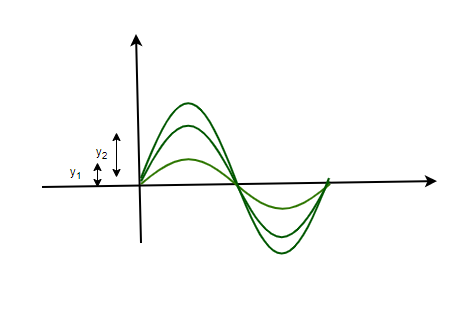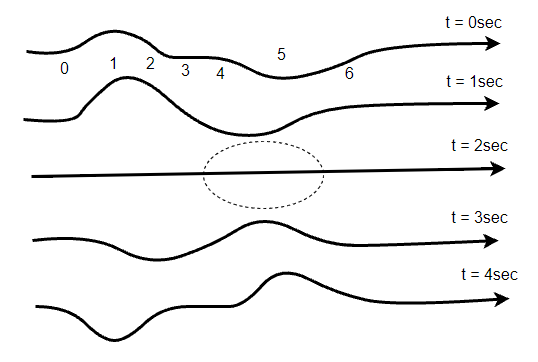# Principle of Superposition of Waves

• Last Updated : 20 Oct, 2021

Waves surround us almost everywhere in real life. The Wifi, Mobile Network, and any other wireless form of communication are comprised of waves of different wavelengths. Due to Thomas Young’s experiments, it was established that light possesses a wave nature. Since it was established that so many physical phenomena around us constitute waves, it became essential to study the superposition between two or more waves. Waves do not behave as  Let’s study these concepts in detail.

### Principle of Superposition

When two or more waves travel in the same medium, they are bound to interact with each other. They retain their wave nature after combining with each other, but usually, the resultant wave is different from both of the individual waves. The superposition principle helps us describe the resulting wave or motion that is produced when two or more waves combine with each other. The figure below shows two waves that are producing some displacement in the particles of the given medium. In this case, the principle of superposition states that,

Attention reader! All those who say programming isn't for kids, just haven't met the right mentors yet. Join the  Demo Class for First Step to Coding Course, specifically designed for students of class 8 to 12.

The students will get to learn more about the world of programming in these free classes which will definitely help them in making a wise career choice in the future.

The resultant displacement of a number of waves in the medium at a particular point is the vector sum of the individual displacements produced by wave at each point.In the figure given above, two waves with individual displacements y1 and y2 are given. Notice that the resultant wave from the superposition of these two waves has a greater displacement than the two individual waves.

The principles of superposition can be applied to any type of waves provided that:

1. The waves that are superimposed are of the same types.
2. The medium in which the waves are propagating behaves linearly, this means that the particles of the medium which are displaced with twice the displacement experience twice the amount of restoring force on them.In the case of waves, the figure above shows two waves that are traveling in opposite directions. These waves produce equal displacements in the rope. Notice that in figure(c) when both waves overlap, the resultant displacement is zero.

In mathematical terms, the superposition principle can be described as given below. Let’s say y1(x, t) and y2(x, t) is the displacements produced by two waves in the medium. Let P be the point where these two ways come and meet. Now using the principle of superposition to find the resultant displacement (y).

y = y1(x, t) + y2(x, t)

If two or more waves are traveling and meeting at one point in a medium and the wave functions for the individual waves are given by,

y = f1(x – vt)

y = f2(x – vt)

…

y = fn(x – vt)

The resultant wave after displacement is given by,

y = f1(x – vt) + f2(x – vt) +  f3(x – vt) + …. fn(x – vt)

### Constructive Interference

Let us consider two waves that are traveling at the same velocity. Since these two waves travel at the same velocity and meet at a particular point. Let’s analyze the resultant amplitude of the wave which originates after the superposition of these waves. Assuming that the equations for displacement produced by both the waves are the same and is given by,

y1 = acos(ωt)

y2 = acos(ωt)

Now using the principle of superposition to find the resultant displacement (y).

y = y1 + y2

⇒ y = acos(ωt) + acos(ωt)

⇒ y = 2acos(ωt)

It’s known that the intensity is proportional to the square of the amplitude. Since amplitude, in this case, becomes twice the original amplitude. The resulting intensity is given by

I = 4I0

Where I0 is the intensity of the original wave.

Since the intensity is increasing after the superposition. This is termed constructive interference.

### Destructive Interference

Let us consider two waves that are traveling at the same velocity. Since these two waves travel at the same velocity and meet at a particular point. Let’s analyze the resultant amplitude of the wave which originates after the superposition of these waves. Assuming that the equations for displacement produced by both the waves are the opposite this time and are given by,

y1 = acos(ωt)

y2 = acos(ωt + 5π

y = y1 + y2

⇒ y = acos(ωt)  + acos(ωt + 5π)

⇒.y = acos(ωt) – acos(ωt)

⇒ y = 0

So, this time two displacements in opposite directions resulted in zero amplitude and zero intensity. This is called destructive interference. The path difference in destructive interference can be generalised to  (n + 0.5)where n = 0, 1, 2, 3 ….

Let’s see some examples of these concepts.

### Sample Problems

Question 1: The phenomena of interference can be explained by which of the following principles:

1. Heisenberg Principle
2. Fermi Principle
3. Superposition Principle
4. Quantum Mechanics

Phenomena of interference can be explained by “Superposition Principle”. It states that,

“The resultant displacement of a number of waves in the medium at a particular point is the vector sum of the individual displacements produced by wave at each point.”

Question 2: Two waves travelling in a medium are given by the following equations,

y1 = 2acos(ωt)

y2 = 2acos(ωt + π

Find the resulting amplitude after their superposition.

y = 2acos(ωt) + 2acos(ωt + π)

⇒ y = 2acos(ωt) – 2acos(ωt)

⇒ y = 0

The resulting amplitude becomes zero.

Question 3: Two waves travelling in a medium are given by the following equations,

y1 = acos(0.5ωt)

y2 = acos(0.5ωt + 2π

Find the resulting amplitude after their superposition.

y = acos(0.5ωt) + acos(0.5ωt + 2π)

⇒ y = acos(0.5ωt) + acos(0.5ωt)

⇒ y = 2acos(0.5ωt)

The resulting amplitude becomes “2a”.

Question 4: Two waves travelling in a medium are given by the following equations,

y1 = 2acos(ωt)

y2 =3acos(ωt + π

Find the resulting amplitude after their superposition.

y = 2acos(ωt) + 3acos(ωt + π)

⇒ y = 2acos(ωt) – 3acos(ωt)

⇒ y = -acos(ωt)

Question 5: Two waves travelling in a medium are given by the following equations,

y1 = acos(ωt)

y2 =acos(ωt + 2π

Find the resulting amplitude after their superposition.